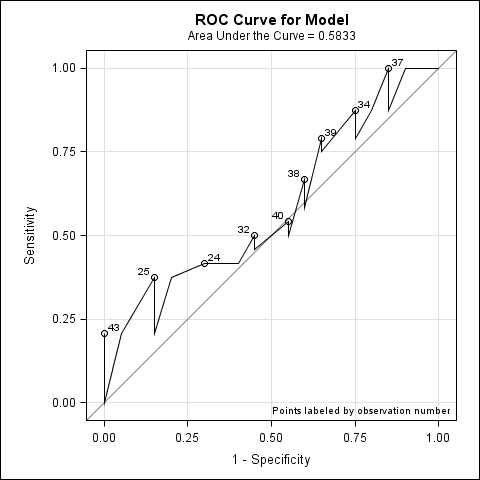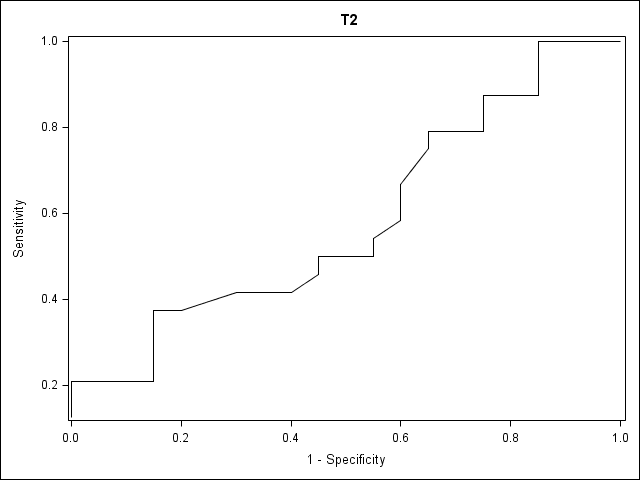## How to fix jagged ROC curves

I'm having an issue with ROC curves when using ODS graphics and proc logistic: namely, the ROC graphs that are automatically generated appear very jagged and improper - see attached for an example. When I produce the ROC curve using proc gplot and the ROC output data generated by proc logistic, everything is fine - the ROC curves are all convex, and the graph doesn't "turn back on itself". Does anyone know why I'm getting these results? Thanks for the help!

Code producing Jagged Curves:

ods graphics on ;

proc logistic data=test_roc plots(only)=(roc(id=obs) effect) descending;

model disease(event='1') = test ;

run;

ods graphics off;

Code producing "nice" curves:

proc logistic data=test_roc descending;

model disease(event='1') = test / outroc=roc_data;

run;

proc gplot data=o1;

plot _sensit_*_1mspec_ /

ctext=black caxis=black vaxis=0 to 1 by .1 haxis= 0 to 1 by .1;

run;

quit;9 REPLIES 9

## Re: How to fix jagged ROC curves

Is this really the program you submitted?  PROC GPLOT appears to be using a different data set (o1) than the output from LOGISTIC (roc_data).

## Re: How to fix jagged ROC curves

Yes, sorry that is was a copy and paste mistake. Here's the proc gplot code:

proc gplot data=roc_data;

plot _sensit_*_1mspec_ /

ctext=black caxis=black vaxis=0 to 1 by .1 haxis= 0 to 1 by .1;

run;

quit;

## Re: How to fix jagged ROC curves

you may want to include both the log and the second curve.

## Re: How to fix jagged ROC curves

Ok will do, here is the log - I made an sgplot to generate the attached image.

805  ods graphics on ;

806  proc logistic data=test_roc plots(only)=(roc(id=obs) effect) descending;

807      model disease(event='1') = test ;

808  run;

NOTE: PROC LOGISTIC is modeling the probability that disease=1.

NOTE: Convergence criterion (GCONV=1E-8) satisfied.

NOTE: There were 44 observations read from the data set WORK.TEST_ROC.

NOTE: PROCEDURE LOGISTIC used (Total process time):

real time           0.70 seconds

cpu time            0.21 seconds

809  quit;

810  ods graphics off;

811

812

813  proc logistic data=test_roc descending;

814       model disease(event='1') = test / outroc=roc_data;

815  run;

NOTE: PROC LOGISTIC is modeling the probability that disease=1.

NOTE: Convergence criterion (GCONV=1E-8) satisfied.

NOTE: There were 44 observations read from the data set WORK.TEST_ROC.

NOTE: The data set WORK.ROC_DATA has 29 observations and 7 variables.

NOTE: PROCEDURE LOGISTIC used (Total process time):

real time           0.03 seconds

cpu time            0.03 seconds

816

817  proc gplot data=roc_data;

818      plot _sensit_*_1mspec_ /

819      ctext=black caxis=black vaxis=0 to 1 by .1 haxis= 0 to 1 by .1;

820  run;

821  quit;

NOTE: There were 29 observations read from the data set WORK.ROC_DATA.

NOTE: PROCEDURE GPLOT used (Total process time):

real time           0.32 seconds

cpu time            0.14 seconds

832

833  proc sgplot data=roc_data;

834      series x=_1mspec_ y=_sensit_;

835  run;

NOTE: PROCEDURE SGPLOT used (Total process time):

real time           0.24 seconds

cpu time            0.07 seconds

NOTE: Listing image output written to SGPlot1.png.

NOTE: There were 29 observations read from the data set WORK.ROC_DATA.## Re: How to fix jagged ROC curves

I don't see anything obvious.  I am concerned that the small sample size may be an issue.  It appears that you only have about 10 non-events, which is smaller than I would use for any logistic regression.

## Re: How to fix jagged ROC curves

The example code I posted uses a "made-up" data set that I generated, but I've had this same problem with real data using sample sizes of about 200. The [proc logistic with ODS graphics] used to work just fine, but something happened and it's now generating these strange ROC curves. i.e. the two methods used to generate the graphs were able to produce the same curves. Not too sure what I did wrong. Thanks for the help though!

## Re: How to fix jagged ROC curves

The ROC curve is supposed to be nondecreasing, so something is wrong.  What version of SAS are you using? Contact SAS Technical Support and send them the data.  I do not see any SAS Notes or Knowledgebase articles that describe this problem.

## Re: How to fix jagged ROC curves

You didn't modify the ODS templates, did you?

## Re: How to fix jagged ROC curves

I was having issues with Java - can't remember the exact errors I was getting - I must've changed something when fixing that. I'll try calling Tech Support and see what they say. Thanks!

Discussion stats
• 9 replies
• 2068 views
• 0 likes
• 3 in conversation